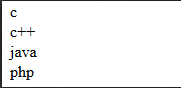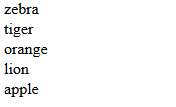# Array sorting in php with example

Array -  Array is a structure in which it stores multiple values in a single  variable. It can be classified into  three types  say  numeric ,associative  and multidimensional array.

Arrays values can be manipulated in different ways..this blog describes how array values are sorted with examples

i) Simple sorting in array

Syntax:  sort(\$variable);

Example:

```//simple sorting of array
//results the data in ascending order by default
\$languages = array("php", "c", "c++", "java");
sort(\$languages);
foreach(\$languages as \$key){
echo \$key;echo "<br>";
}
```

Output:`ii)Sorting the array values  in reverse order`

Syntax:  rsort(\$variable);

Example:

```//sorting the values in reverse order
\$array_rsort =  array("apple", "zebra", "orange" , "lion" ,"tiger");
rsort(\$array_rsort);
foreach(\$array_rsort as \$key){
echo "\$key";
echo "<br>";
}
```

Output:Category: